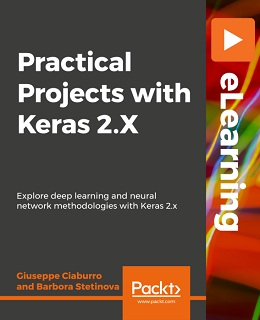# Practical Projects with Keras 2.x [eLearning]### Practical Projects with Keras 2.x [eLearning]

##### English | MP4 | AVC 1920×1080 | AAC 48KHz 2ch | 2h 47m | 3.30 GB eLearning | Skill level: All Levels

Practical Projects with Keras 2.x [eLearning]: Through engaging and elaborate projects, gain the knowledge and skills to implement neural networks and deep learning techniques using Keras

Keras is a user-friendly, modular, and intuitive neural network library that enables you to experiment with deep neural networks.

Practical Projects with Keras 2.x explains how to leverage the power of Keras to build and train state-of-the-art deep learning models through a series of practical projects that look at a range of real-world application areas. You’ll begin by exploring concepts underlying regression, such as the differences between simple and multiple regression and algebraically representing a multiple linear regression problem. Moving on, you’ll discover various classification techniques, such as Naive Bayes and Mixture Gaussian, and use these to solve practical problems. The course ends by teaching you the basic concepts of multilayer neural networks and how to implement them in Keras environment.

• Apply regression methods to your data and understand how the regression algorithm works
• Import and organize data for neural network classification analysis
• Solve a regression problem through the least squares algorithm
• Use a classification algorithm to predict the outcome of an event
• Train, test, and deploy a model in Keras environment
• Implement multilayer neural networks in Keras
• Improve the performance of a model by removing outliers

By the end of this course, you will have the knowledge you need to train your own deep learning models to solve different kinds of problems.